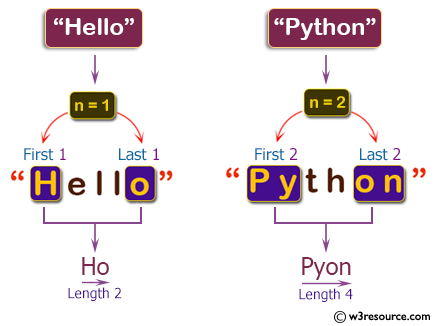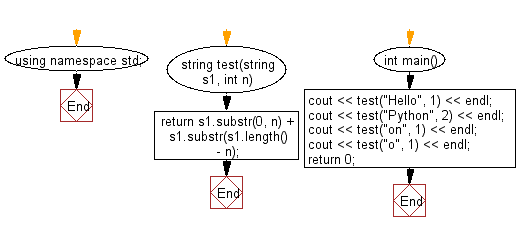﻿ C++ : New string of first and last n characters of a string# C++ Exercises: Create a new string using the first and last n characters from a given string of length at least n

## C++ Basic Algorithm: Exercise-68 with Solution

Write a C++ program to create a new string using the first and last n characters from a given string of length at least n.

Sample Solution:

C++ Code :

``````#include <iostream>

using namespace std;

string test(string s1, int n)
{
return s1.substr(0, n) + s1.substr(s1.length() - n);
}

int main()
{
cout << test("Hello", 1) << endl;
cout << test("Python", 2) << endl;
cout << test("on", 1) << endl;
cout << test("o", 1) << endl;
return 0;
}
``````

Sample Output:

```Ho
Pyon
on
oo
```

Pictorial Presentation:Flowchart:C++ Code Editor: